### Decision Tree Introduction with Examples

• date 28th January, 2020 |
• by Prwatech |

# Decision Tree Introduction with Examples

Decision Tree Introduction with examples, Are you the one who is looking forward to knowing Decision tree Introduction with Examples? Or the one who is looking forward to knowing types of decision tree algorithm in Machine Learning and How to create Decision Tree or Are you dreaming to become to certified Pro Machine Learning Engineer or Data Scientist, then stop just dreaming, get your Data Science certification course with Machine Learning from India’s Leading Data Science training institute. A Decision tree has analogies in real life and influenced both classification and regression in Machine Learning. A decision tree represents decisions and decision making visually. In this blog, we will learn types of Decision tree Introduction, types of decision tree algorithm, How to create Decision Trees, Advantages, and Disadvantages of Decision Tree. Do you want to know Steps for Decision tree using Python in machine learning, So follow the below-mentioned Decision tree Introduction with examples in Machine Learning from Prwatech and take advanced Data Science training like a pro from today itself under 10+ Years of hands-on experienced Professionals.

## Decision Tree in Machine Learning

The decision tree is the most influential and popular tool for classification and prediction. A decision tree is a structure in which each interior node signifies a test on a feature, each leaf node indicates a class label and branches signify combinations of features that lead to those class labels. The paths from the root to the leaf indicate classification rules. The decision tree algorithm falls under the type of supervised learning. It can be applied in cases of both regression and classification problems. A tree representation is used by a Decision tree to solve a problem where every leaf node resembles a class label and attributes represent the internal node of a tree.

### Common terms used with Decision trees: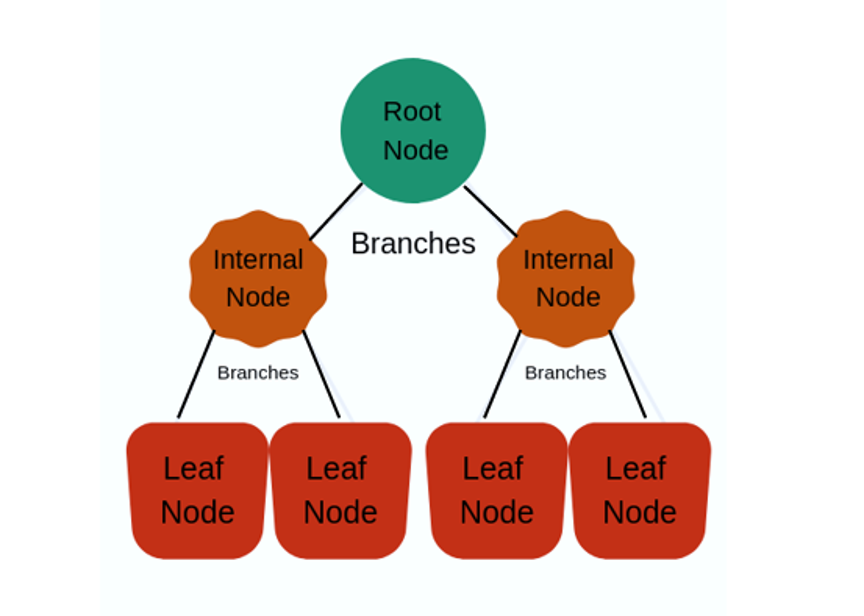Root Node: It signifies the whole population or sample which is further divided into two or more homogeneous sets. Splitting: It is a method of dividing a node into two or more sub-nodes. Decision Node: A sub-node divided into further sub-nodes, then it is called a decision node. Leaf/ Terminal Node: Nodes that do not divide into sub-nodes are called Leaf or Terminal node. These are end nodes. Pruning: The process of removing sub-nodes from the decision node is called pruning. It’s exactly opposite to splitting. Branch / Sub-Tree: A subpart of the entire tree is called branch or sub-tree. Parent and Child Node: A node,  that is divided into sub-nodes is known as the parent node of sub-nodes. The sub-nodes are the child of the parent node.

## How to create a Decision Tree

While creating a decision tree, on every node of a tree we have to ask a different types of questions. Based on the asked question we will calculate the information gain corresponding to it.

### Entropy:

Entropy is a measure of uncertainty of a random variable. It symbolizes the impurity of a random collection of examples. The greater entropy indicates higher information content.

### Information Gain:

It is the entity that is required to decide which feature is to split or divide it on at every step in building the tree. To keep tree small, at every step we should select the split that outcomes in the purest child nodes. Information is a commonly used measure of purity. Information value measures the quantity of information, a feature giving about the class. The field has the highest information gain will be taken as the main field to split the whole dataset. This process will continue until all children nodes are pure, or until the information gain is 0. Usually, decision tree creation works top-down. It selects a variable at each step that best splits the set of items. Different algorithms follow different matrices for measuring best. Suppose X is a set of instances, P is an attribute, Xv is the subset of X with P = v, and Values (P) is the set of all possible values of P, then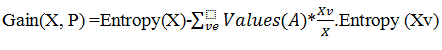### Information Gain Example:

For the set X = {a,a,b,b,b,b,a,b,a,b} Total instances   = 10 The occurrence of  ‘a’  = 4 The occurrence of  ‘b’  = 6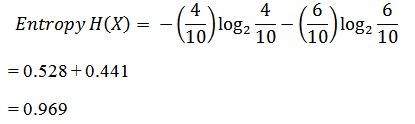### Gini Impurity

It is a calculation of the probability of an inaccurate classification of a new instance for a random variable if the new instance is randomly classified according to the distribution of class labels from the data set. If the dataset is pure then the probability of incorrect classification is 0. If the input sample is a mixture of a variety of classes then the likelihood of inaccurate classification will be high.

## Decision Tree Algorithm Example:

Let’s take a weather report into consideration for classifying on basis of different categories. The target here is to decide whether to play cricket or not for a particular day.
 Day Weather Temperature Humidity Wind 1 Sunny Hot High Weak 2 Cloudy Hot High Weak 3 Sunny Mild Normal Strong 4 Cloudy Mild High Strong 5 Rainy Mild High Strong 6 Rainy Cool Normal Strong 7 Rainy Mild High Weak
Now based on the Decision tree hierarchy, first we have to find here, which feature is the root node. Here we have to calculate ‘Entropy’ for that feature. It can be calculated as: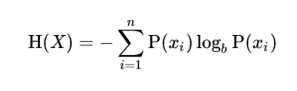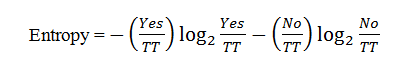Where TT= Total number of targets=14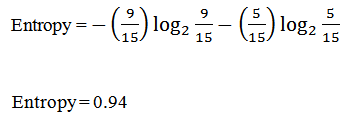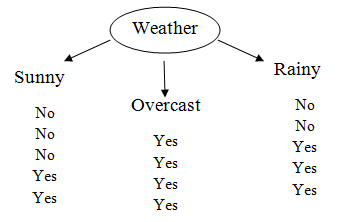First, we check for the feature ‘Weather’. This image shows the distribution of the target variable depending on subcategories. It is having three subcategories as ‘Sunny’, ‘Overcast’, and ‘Rainy’. We will first calculate individual entropy for each subcategory. It is giving target value yes or No as follows: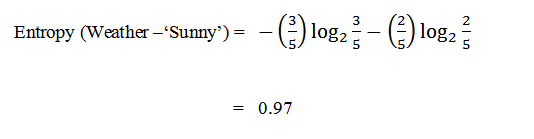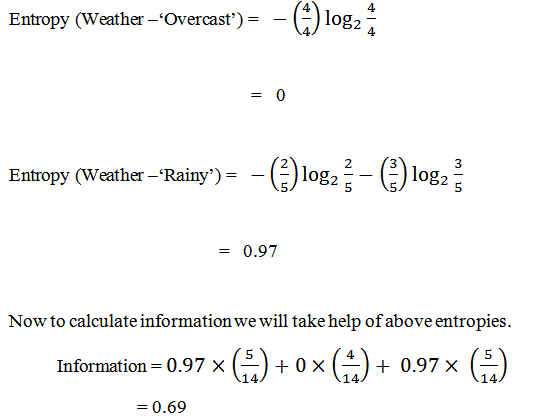It is information for ‘Weather’. To check the information gain we have to subtract information from total entropy. Information gain = Entropy – information = 0.94 – 0.69                                      = 0.25 Like this, if we calculate the information gain for all features we get: Information Gain for temperature = 0.03 Information Gain of Humidity = 0.152 Information Gain of Wind = 0.048 It is clearly observed that from the calculation, the information gain of Weather is highest among all. So ‘Weather’ will be the root node. After this, the table is modified by excluding the column of Weather. And the procedure is repeated to get the next internal or leaf nodes. Those whose information gain is very negligible are eliminated. Finally, we get a structure of a decision tree like this: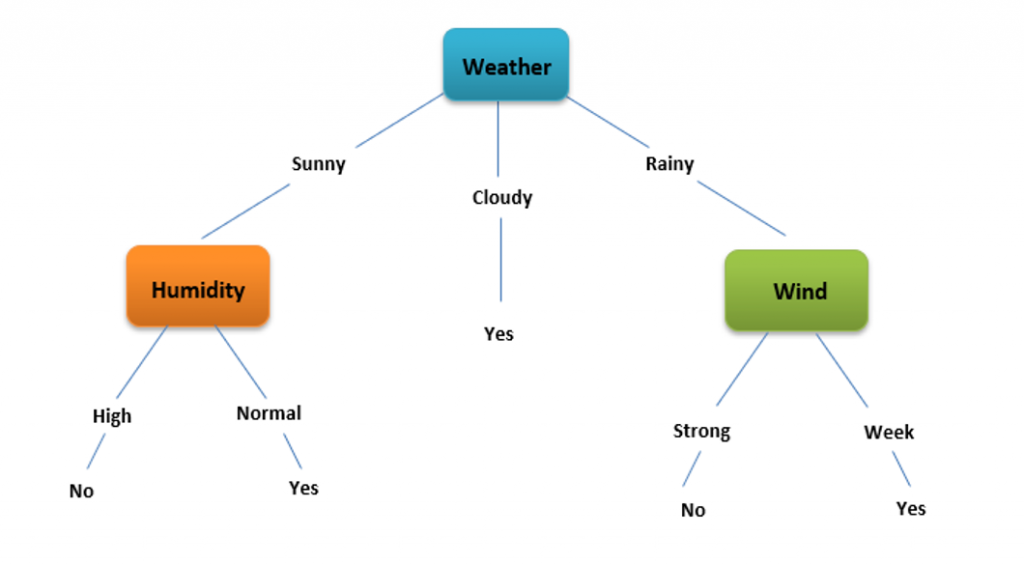## Types of Decision Tree Algorithm

Decision trees are created using an algorithmic approach that determines ways to split a data set based on different conditions. It is one of the most commonly used and practical methods for supervised learning. Decision Trees are a non-parametric supervised learning method utilized for both classification and regression tasks. Classification Tree: Decision tree models where a target variable can take a discrete set of values are called classification trees. Regression Tree: These are decision trees with the target variable having continuous values (typically real numbers) are called regression trees. The general term for this is the Classification and Regression Tree (CART).

It is easy to implement and understand. It can handle both classification and regression data. It provides resistance to outliers. For that some data pre-processing is necessary.

It disposes to overfitting. It requires some kind of measurement for performance. It is necessary to handle parameter tuning correctly.

### To avoid overfitting the Decision tree model

Overfitting is a key problem for every model in machine learning. The new sample test will be poorly generalized if the model is overfitted. To avoid the issue of overfitting in decision trees we prefer to remove the branches that make use of features having low significance. This method is known as pruning or post-pruning. Using this method, the complexity of the tree is reduced so that it recovers predictive accuracy by the reduction of overfitting. Pruning should reduce the size of the learning tree without affecting predictive accuracy as measured by a cross-validation set. There are two major Pruning techniques. Minimum Error: The tree is trimmed back to a point where the cross-validated error is a least or minimum. Smallest Tree: The tree is trimmed back, slightly next to minimum error. Means this pruning i.e. trimming generates a decision tree with cross-validation error within 1 standard error of the minimum error.

## Steps for Decision tree using Python

Import Library import numpy as np import pandas as pd from sklearn.ensemble import ExtraTreesClassifier import matplotlib.pyplot as plt Import the data set. data = pd.read_csv("Your File Path") Divide the data set into independent and dependent parts. X = data.iloc[:,0:20]  #independent columns y = data.iloc[:,-1] #dependent columns Perform feature selection. Perform Label Encoding if the data set contains any string values. Check whether the data set contains any null values or not. data.isna().any() Perform different Data Cleaning operations to remove null values. Split the data set into two parts for training and testing. from sklearn.model_selection import train_test_split X_train, X_test, y_train, y_test = train_test_split(X, y, random_state = 47, test_size = 0.33) Import Decision Tree from sklearn from sklearn. tree import DecisionTreeClassifier Create tree object and train the model using the training sets and check score model = tree.DecisionTreeClassifier(criterion=’gini’) # Here we can change the algorithm as gini or entropy by default it is gini for classification. model = tree.DecisionTreeRegressor() for regression model.fit(X, y) model.score(X, y) Predict Output and calculate the accuracy percentage of the model created. predicted = model.predict(x_test) from sklearn.metrics import accuracy_score print('Accuracy Score on train data: ', accuracy_score(y_true=y_train, y_pred=clf.predict(X_train))*100) print('Accuracy Score on test data: ', accuracy_score(y_true=y_test, y_pred=y_pred)*100). We hope you understand the Decision tree Introduction with examples in Machine Learning concepts and types of decision tree algorithm, Advantages of Decision Tree, Disadvantages of Decision Tree, and Steps for Decision tree using Python. Get success in your career as a Data Scientist/ Machine Learning Engineer by being a part of the Prwatech, India's leading Data Science training institute in Bangalore.

### Quick Support